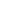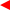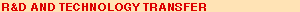contentsERCIM News No.50, July 2002# Random Indexing of Linguistic Units for Vector-Based Semantic Analysis

by Magnus Sahlgren

The Stochastic Pattern Computing project at SICS studied the mathematical foundations of human-like flexible information processing methods that compute with high-dimensional random vectors. The project ended in 2001 and led to the development of the Random Indexing technique for acquiring and representing semantic information about linguistic units.

Vector-space models have been used for over a decade to automatically acquire and represent semantic information about words, documents and other linguistic units. In short, the idea is to use co-occurrence information to construct a multi-dimensional semantic space in which the linguistic units are represented by vectors whose relative distances represent semantic similarity between the linguistic units. The space is constructed by collecting co-occurrence information in a words-by-contexts frequency matrix where each row represents a unique word and each column represents a context (typically a word or a document). The cells of the co-occurrence matrix records the (normalised) frequency of co-occurrence with the given context.

The rows (and the columns) of the frequency matrix can be interpreted as multi-dimensional context vectors where the elements are (normalised) frequency counts, and the dimensionality is the number of contexts in the text data. Thus, the representations are local. Now, the inherent problem with using local representations in natural language processing is that the size, or dimensionality, of the representations will grow with the size of the data. This means that the model will not scale very well, and that the co-occurrence matrix will soon become computationally intractable when the vocabulary and the document collection grows. To make the method practically feasible, it is necessary to reduce the dimensionality of the matrix.Same catch, different languages. The Random Indexing technique can be used to find similar terms across languages. This can be used for example when searching for information on the World Wide Web.

However, dimension reduction techniques tend to be computationally very costly, which means that if efficiency is important, it may not be practicable to use such techniques. Furthermore, dimension reduction is a one-time operation, with a rigid result, which means that new data cannot be added to the model once a dimension reduction has been performed. As an alternative to vector-space models that use local co-occurrence matrices and some form of dimension reduction, we have studied the use of distributed representations that eliminates the need for separate dimension reduction of the co-occurrence matrix. The technique, which we call Random Indexing, accumulates a words-by-contexts co-occurrence matrix by incrementally adding together distributed representations in the form of high-dimensional (ie on the order of thousands) sparse random index vectors. The index vectors contain a small number of non-zero elements, which are either +1 or -1, with equal amounts of both. For example, if the index vectors have eight non-zero elements in, say, 1,800 dimensions, they have four +1s and four -1s.

The index vectors serve as indices or labels for words or documents, depending on which kind of co-occurrences we want to use. When using document-based co-occurrences, the documents are represented by high-dimensional sparse random index vectors, which are used to accumulate a words-by-contexts matrix by the following procedure: every time a given word occurs in a document, the document’s index vector is added to the row for the word in the matrix. The procedure is similar when using word-based co-occurrences: first, we assign a high-dimensional sparse random index vector to each word type in the data. Then, every time a given word occurs in the data, the index vectors of the surrounding words are added to the row for the focus word. Words are thus represented in the co-occurrence matrix by high-dimensional context vectors that contain traces of every context (word or document) that the word has co-occurred with (or in).

Note that the same procedure will produce a local frequency matrix if we use unary vectors of the same dimensionality as the number of documents (when using document-based co-occurrences) or the size of the vocabulary (when using word-based co-occurrences). These index vectors would have a single 1 marking the place of the context (word or document) in a list of all contexts - the nth bit of the index vector for the nth document or word would be 1. Mathematically, the unary local vectors are orthogonal, whereas the random index vectors are only nearly orthogonal. However, since there exist a much larger number of nearly orthogonal than truly orthogonal directions in a high-dimensional space, choosing random directions gets us sufficiently close to orthogonality to provide an approximation of the unary vectors. The amount of noise we introduce by choosing random directions is so small that it does not have any noticeable effect on the similarity relations between the entries, which means that the local frequency matrix and the Random Indexing matrix contain approximately the same information. By using, for example, 1,800-dimensional random index vectors with 8 non-zero elements, we may accumulate approximately the same co-occurrence information in a 50,000 by 2,000 matrix (assuming a vocabulary of 50,000 words in 30,000 documents) as we do in a 50,000 by 50,000 or 50,000 by 30,000 matrix using local representations for word-based vs. document-based co-occurrences.

By using the random index vectors to accumulate the co-occurrence matrix, we effectively perform a dimension reduction of the data, without the need for an explicit dimension reduction phase. This makes Random Indexing more efficient than techniques using computationally heavy dimension reduction techniques. It also makes the technique more flexible towards new data, since it may be immediately added to the model without the need to recompute the entire matrix - a new word only needs a new row in the co-occurrence matrix, and existing context vectors may simply be updated with the new information. Furthermore, the high-dimensional sparse random index vectors may be used to cover basically any size of the vocabulary, without the need to increase the dimensionality of the vectors, which makes the technique extremely scalable.

The Random Indexing approach thus presents an attractive alternative to vector-space models using local representations and computationally heavy dimension reduction techniques.